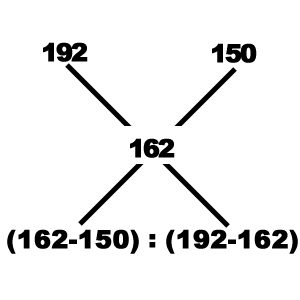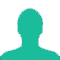# The ratio, in which tea costing Rs. 192 per kg is to be mixed with tea costing Rs. 150 per kg so that the mixed tea when sold for Rs. 194.40 per kg, gives a profit of 20%.

A. 2 : 5

B. 3 : 5

C. 5 : 3

D. 5 : 2

### Solution(By Examveda Team)

CP of first tea = Rs. 192 per kg.
CP of Second tea = Rs. 150 per kg.
Mixture is to be sold in Rs. 194.40 per kg, which has included 20% profit. So,
SP of Mixture = Rs. 194.40 per kg.
Let the CP of Mixture be Rs. X per kg. Therefore,
X + 20% of X = SP
$$\frac{{6{\text{X}}}}{5}$$ = 194.40
6X = 194.40 *5
X = Rs. 162 per kg.
Let N kg of first tea and M kg of second tea to be added.
Now, Using Alligation,
We get,$$\frac{{\text{N}}}{{\text{M}}} = \frac{{12}}{{30}}$$
N : M = 2 : 5

1.The formula is d-m/m-c . So the ans should be 5:2

2.162

3.192x+150y=194.4(x+y)×100/120
1152x+900=972x+972y
180x=72y
x:y=2:5

4.CP of mixture = 194.40*(5/6) = 162
Applying rule of Alligation :
192 ································ 150
················· 162 ····················
12 : 30 or 2 : 5 (Ans.)

5.Price of tea A=192 per kg
Price of tea B=150 per kg
If mixture is sold at price 194.40 per kg then you will get 20% profit.
So calculate cost price of mixture.
The equation will be like-

Let the price of mixture= X
Means, you will get 20% profit of X.
Then:
X + (20/100 *X)=194.40
After solving this equation
The Cost price of mixture will be 162 per kg (approx).
Now have a look on the explaination given above to solve this question.

6.From this trick we learn that if we are taking c.p. then c.p. is used in whole quest.

7.8.sir solution anupaat kaise devide kiya
Plz step by step solution bateyn thanks

9.kaise hua hai ye

10.11.